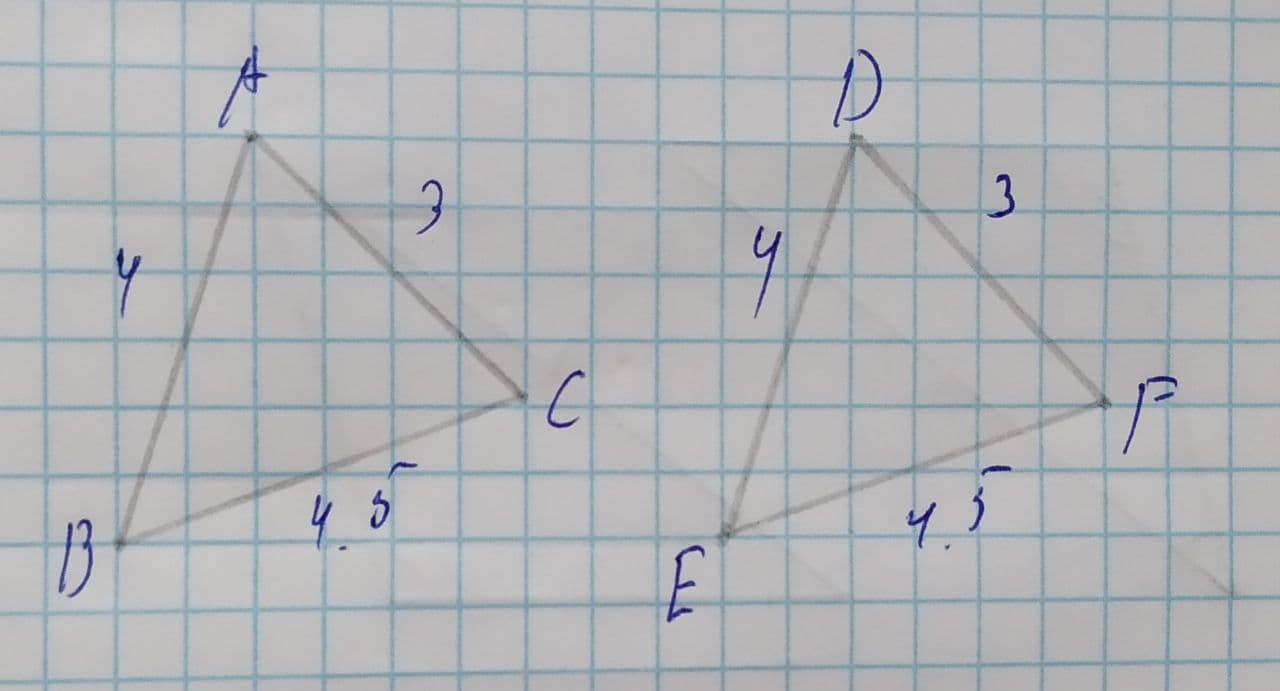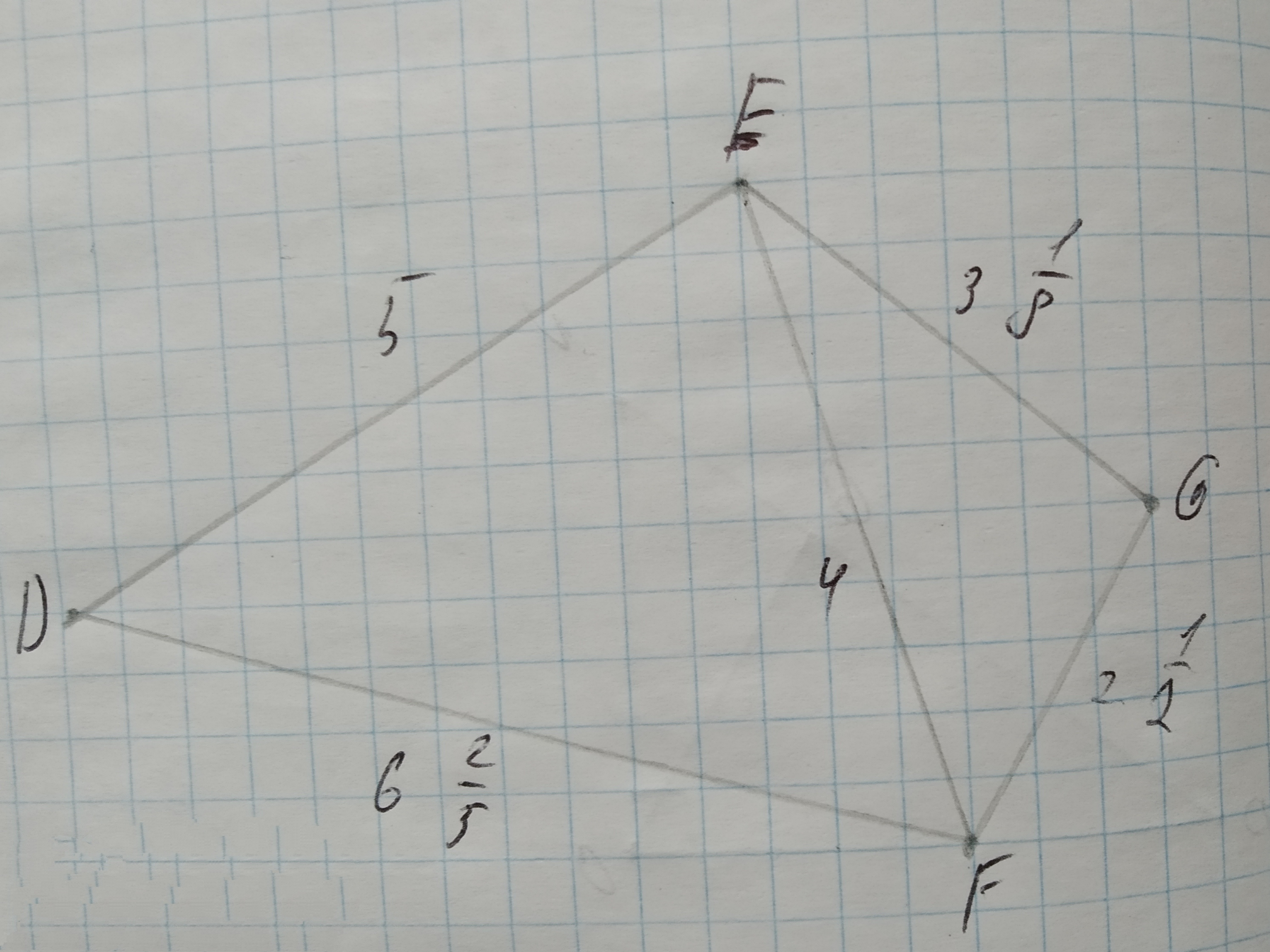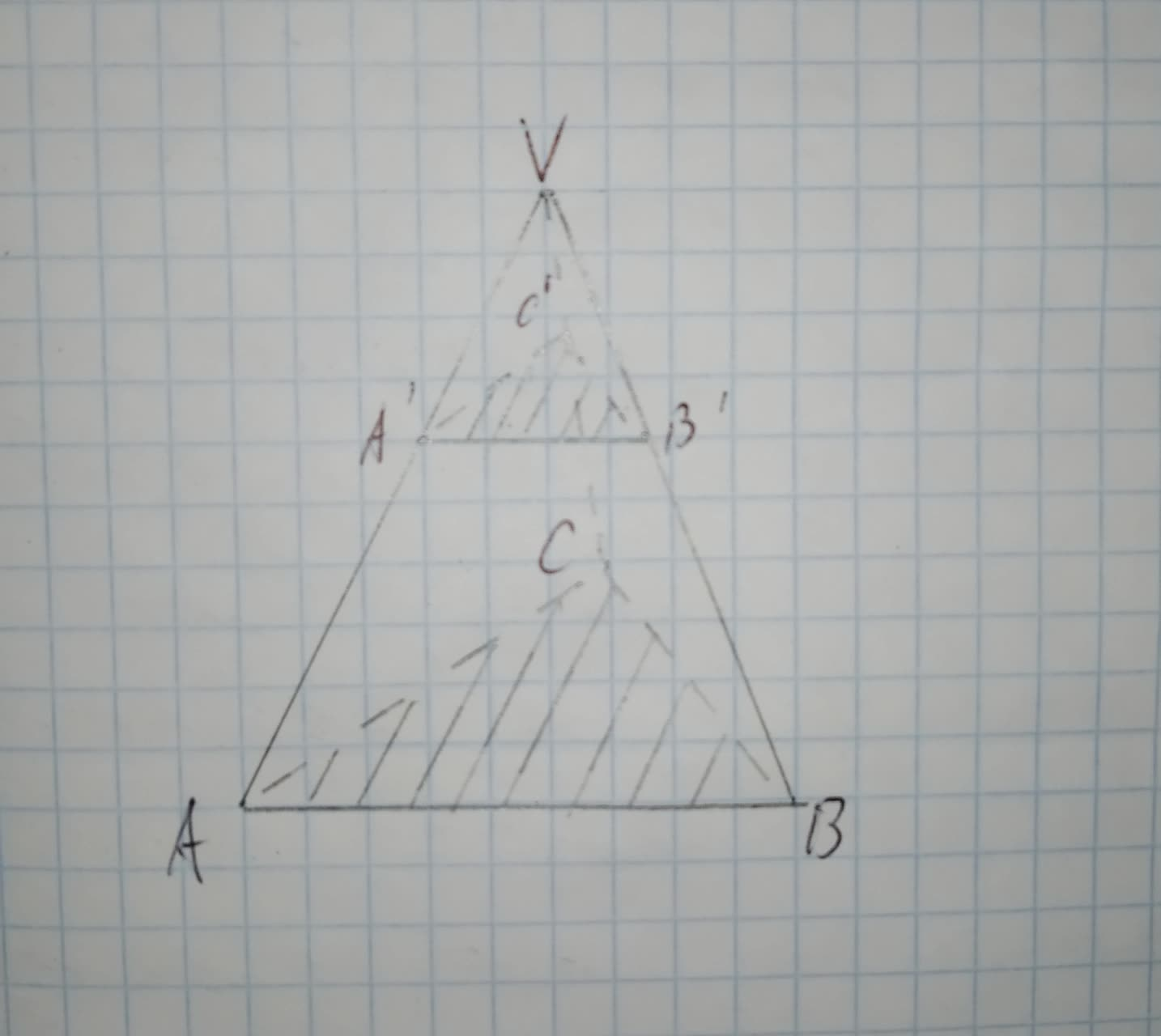Ask question

# To determine: The ratio of the sides of the triangles ABC and GHI. Given: Triangle ABC that is 75% of its corresponding side in triangle DEF. Triangle GHI that is 32% of its corresponding side in triangle DEF.# To determine: The ratio of the sides of the triangles ABC and GHI. Given: Triangle ABC that is 75% of its corresponding side in triangle DEF. Triangle GHI that is 32% of its corresponding side in triangle DEF.

Question
Similarityasked 2021-03-09
To determine: The ratio of the sides of the triangles ABC and GHI.
Given:
Triangle ABC that is 75% of its corresponding side in triangle DEF.
Triangle GHI that is 32% of its corresponding side in triangle DEF.

## Answers (1)2021-03-10
Calculation:
Since, the side of the triangle $$\displaystyle\triangle{A}{B}{C}$$ is 75% of the side of the triangle $$\displaystyle\triangle{D}{E}{F}$$.
$$\displaystyle\frac{{{A}{B}}}{{{D}{E}}}=\frac{{{75}}}{{{100}}}$$
$$\displaystyle{A}{B}=\frac{{3}}{{4}}{D}{E}$$...(1)
and, the side of the triangle $$\displaystyle\triangle{G}{H}{I}$$ is 32% of the side of the triangle $$\displaystyle\triangle{D}{E}{F}$$.
$$\displaystyle\frac{{{G}{H}}}{{{D}{E}}}=\frac{{{32}}}{{{100}}}$$
$$\displaystyle\frac{{{G}{H}}}{{{D}{E}}}=\frac{{{8}}}{{{25}}}$$
$$\displaystyle{D}{E}=\frac{{{25}}}{{{8}}}{G}{H}$$...(2)
Substitute equation (1) in equation (2).
$$\displaystyle{A}{B}=\frac{{3}}{{4}}{\left(\frac{{{25}}}{{{8}}}{G}{H}\right)}$$
$$\displaystyle\frac{{{A}{B}}}{{{G}{H}}}=\frac{{{75}}}{{{32}}}$$
Therefore, the ratio of the sides of the triangles ABC and GHI is $$\displaystyle\frac{{{75}}}{{{32}}}$$.

### Relevant Questionsasked 2021-03-02
To determine: Whether the triangle ABC and GHI are similar to each other.
Given:
Triangle ABC that is 75% of its corresponding side in triangle DEF.
Triangle GHI that is 32% of its corresponding side in triangle DEF.asked 2021-05-16
To prove : The similarity of $$\displaystyle\triangle{N}{R}{T}$$ with respect to $$\displaystyle\triangle{N}{S}{P}$$.
Given information: Here, we have given that $$\displaystyle\overline{{{S}{P}}}$$ is altitude to $$\displaystyle\overline{{{N}{R}}}\ {\quad\text{and}\quad}\ \overline{{{R}{T}}}$$ is altitude to $$\displaystyle\overline{{{N}{S}}}$$.asked 2021-02-21
To prove: The similarity of $$\displaystyle\triangle{N}{W}{O}$$ with respect to $$\displaystyle\triangle{S}{W}{T}$$.
Given information: Here, we have given that NPRV is a parallelogram.asked 2021-04-16
To prove: The similarity of $$\displaystyle\triangle{B}{C}{D}$$ with respect to $$\displaystyle\triangle{F}{E}{D}$$.
Given information: Here, we have given that $$\displaystyle\overline{{{A}{C}}}\stackrel{\sim}{=}\overline{{{A}{E}}}\ {\quad\text{and}\quad}\ \angle{C}{B}{D}\stackrel{\sim}{=}\angle{E}{F}{D}$$asked 2021-04-15
A car initially traveling eastward turns north by traveling in a circular path at uniform speed as in the figure below. The length of the arc ABC is 235 m, and the car completes the turn in 33.0 s. (Enter only the answers in the input boxes separately given.)
(a) What is the acceleration when the car is at B located at an angle of 35.0°? Express your answer in terms of the unit vectors $$\displaystyle\hat{{{i}}}$$ and $$\displaystyle\hat{{{j}}}$$.
1. (Enter in box 1) $$\displaystyle\frac{{m}}{{s}^{{2}}}\hat{{{i}}}+{\left({E}{n}{t}{e}{r}\in{b}\otimes{2}\right)}{P}{S}{K}\frac{{m}}{{s}^{{2}}}\hat{{{j}}}$$
(b) Determine the car's average speed.
3. ( Enter in box 3) m/s
(c) Determine its average acceleration during the 33.0-s interval.
4. ( Enter in box 4) $$\displaystyle\frac{{m}}{{s}^{{2}}}\hat{{{i}}}+$$
5. ( Enter in box 5) $$\displaystyle\frac{{m}}{{s}^{{2}}}\hat{{{j}}}$$asked 2021-04-17

State the congruence relation for $$\displaystyle\triangle{A}{B}{C}\ {\quad\text{and}\quad}\ \triangle{D}{E}{F}$$.A.SSS
B.SSA
C.AAA
D.SASasked 2020-10-27
Given $$\displaystyle\triangle{D}{E}{F}{\quad\text{and}\quad}\triangle{E}{G}{F}$$ in the diagram below, determine if the triangles are similar. If so, write a similarity statement, and state the criterion used to support your claim.asked 2021-05-12
To find: The triangle congruence criteria to prove triangles ABC and ADC are congruent.asked 2020-10-31
Determine whether $$\displaystyle\triangle{A}{B}{C}{\quad\text{and}\quad}\triangle{D}{E}{F}$$ are similar for each set of measures. If so, identify the similarity criterion.
1.$$\displaystyle{m}\angle{A}={20},{m}\angle{C}={20},{m}\angle{D}={40},{m}\angle{F}={40}$$
2.$$\displaystyle{m}\angle{A}={20},{m}\angle{C}={4}{u},{m}\angle{D}={20},{m}\angle{F}={40}$$
3.$$\displaystyle{A}{B}={20},{B}{C}={40},{m}\angle{B}={53}$$,
$$\displaystyle{D}{E}={10},{E}{F}={20},{m}\angle{E}={53}$$asked 2021-02-02
To determine:To Find:the measure of side BB' in the figure shown such that VA' = 15, A'A=20 and A'B'=24
Given:
Figure is shown below....